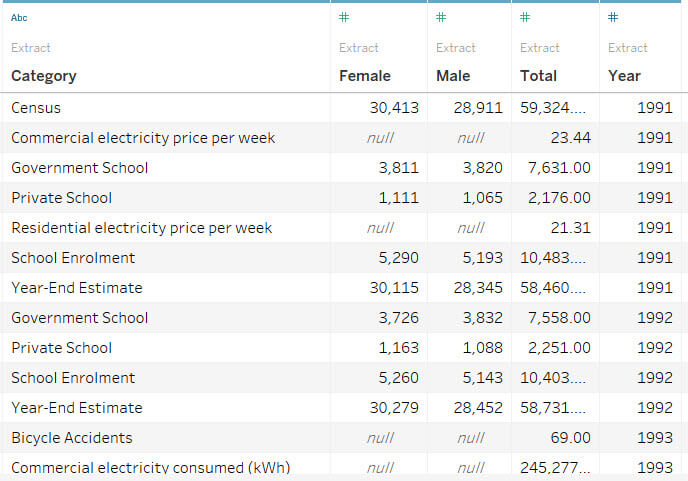## Tableau Tip: Return the value of a single dimension member

by Naledi Hollbruegge

This is a technique I struggled with until fairly recently and my Tableau skills have improved a lot since I learned how to write these calculations. Basically, for some datasets or chart types it is useful to be able to isolate just one member of a dimension and a particular value for it. You can think of it as unpivoting a table.

For example, a few weeks ago Andy posted his Makeover Monday challenge on a Bermuda dataset. This was set up with just five columns. The category, the year, and then the values for males, females, and the total. So when you include the category dimension then this will show all the categories. This could be described as a ‘long’ dataset, where you have a lot of rows and few columns. It could also be presented as a ‘wide’ table, with each category as a separate column.

One way to deal with a dataset like this is to filter out those categories that you are not interested in, but this approach can lead to issues with some chart types as you are filtering these categories at the view level and thus excluding them completely. This gives you very limited control over your fields.The approach I would suggest in this instance is to create a calculated field for each of the categories that you want to use. Thus, we are extracting a member of the dimension ‘category’ and a measure connected with it.

Let’s say we want to build a bullet chart, showing a bar for the Total for the category “Private School” and a Gantt bar for the Total for the category “Government School”. This isn’t possible with the first technique since we only have one measure.

In order to isolate our dimension members we create two calculated fields as follows:

IF [Category] = ‘Government School’ then [Total] END

IF [Category] = ‘Private School’ then [Total] END

Make sure you type your dimension member names exactly as they are spelled in the dataset. In each case, the calculation returns just the value of Total for the specific named category, so basically we are filtering out just the information we want for this field. You could also define these further, to just show the value for one year, for instance adding AND [Year] = ‘1991’ which would then just return the total value in that category in the year 1991. The downside of this approach is of course that we require a new calculation for each dimension member and each associated value. So if we wanted to compare the total values to those of each gender we would also have to make four new calculated fields, again specifying each category and then the measures ‘Female’ and ‘Male’.

Now that we have two separate measures we can build our bullet graph by making a dual axis as shown in the video below.

This is just one example of when it is useful to create a new calculated field to return just the value for one category. Understanding how to do this helped me better understand how Tableau processes data and enabled me to build more complex and flexible visualisations.Naledi Hollbruegge

Thu 25 Aug 2016

Tue 23 Aug 2016

Mon 15 Aug 2016